Ask your WordPress questions! Pay money and get answers fast!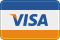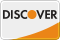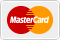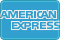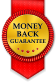# display a specific category level of a post inside the loop #2 WordPress

• SOLVED

The person who answered refused to didn't want to fix it, so i'm now asking for this code to be edited so that it works if there is more one or more than than 1 child category per category. If there is 2 or more children then display the categories in a simple list.

2014-02-22

put in functions.php of your theme
``` function get_level(\$category, \$level = 0) { if (\$category->category_parent == 0) { return \$level; } else { \$level++; \$category = get_category(\$category->category_parent); return get_level(\$category, \$level); } } function display_cat_level( \$level = 0 , \$link=false ){ \$cats = get_categories( array( 'hide_empty' => 0 ) ); if( \$cats ){ foreach(\$cats as \$cat){ \$current_cat_level = get_level(\$cat); if( \$current_cat_level == \$level ){ if(\$link==true) { echo '<a href="'.get_category_link(\$cat->cat_ID).'">'.\$cat->name."</a><br />"; } else { echo \$cat->name."<br />"; } } } } } ```

put where you want output with link of 3rd level categories
remember 0 is the first level so 0 will display main category
1 will display child of main category and so on. In sort level starts with 0.
``` display_cat_level(3,true); ```
if want content without link then just give level.
``` display_cat_level(3); ```

Doesn't work... displays every child cat of every parent cat!! You might like to test it first.

You mean you want sub level categories of particular parent category?

``` function get_level(\$category, \$level = 0) { if (\$category->category_parent == 0) { return \$level; } else { \$level++; \$category = get_category(\$category->category_parent); return get_level(\$category, \$level); } } function display_cat_level( \$level = 0 , \$link=false, \$parent = NULL ){ \$catArgs = array( 'hide_empty' => 0 ); if( \$parent != NULL ){ \$catArgs['child_of'] = \$parent; } \$cats = get_categories( \$catArgs ); if( \$cats ){ foreach(\$cats as \$cat){ \$current_cat_level = get_level(\$cat); if( \$current_cat_level == \$level ){ if(\$link==true) { echo '<a href="'.get_category_link(\$cat->cat_ID).'">'.\$cat->name."</a><br />"; } else { echo \$cat->name."<br />"; } } } } } ```